Math & Beyond
How to solve Questions on Mirror and Water Images?
34.5k views

 1 Introduction 2 What is a Mirror Image? 3 What is Water Image? 4 Example Problems 5 Practice Problems 6 Conclusion 7 Frequently Asked Questions (FAQs)

07 January 2021

## Introduction

As children, we have fond memories of playing a dress-up game in front of a mirror. Some of us have also visited our grandparents’ place and went out playing with our friends by the water bodies.

We used to look into the water and think that there is another person looking at us from underneath the water.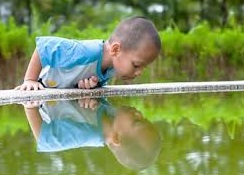Have we ever realized that there is a certain degree of the reasoning behind these concepts? These concepts are used to test the reasoning capability of a child in various aptitude tests.

## How to solve Questions on Mirror and Water Images-PDF

Here is a PDF where u can find Mirror and Water Images examples and practice questions in the form of PDF. Here is a downloadable PDF to explore more.

### A Tale about Monu and Sita

Monu and Sita are two students who are studying in Grade 6. They are preparing for the Olympiad which will be conducted in the month of November.

They were very confused about the concepts of mirror and water images and so they approached their Maths teacher, Anita ma'am, to get their doubts clarified.

Anita ma'am understood their doubts and started explaining them in the following manner to help Monu and Sita understand the concepts clearly:

• What is a Mirror Image?
• What is Water Image?
• Problems involving Mirror images and Water images with solutions.

## What is a Mirror Image?

The Mirror Image which we see in a plane mirror is a reflection of an object which will appear almost identical to the object but will be reversed in the manner in such a way that the image is exactly perpendicular to the plane mirror.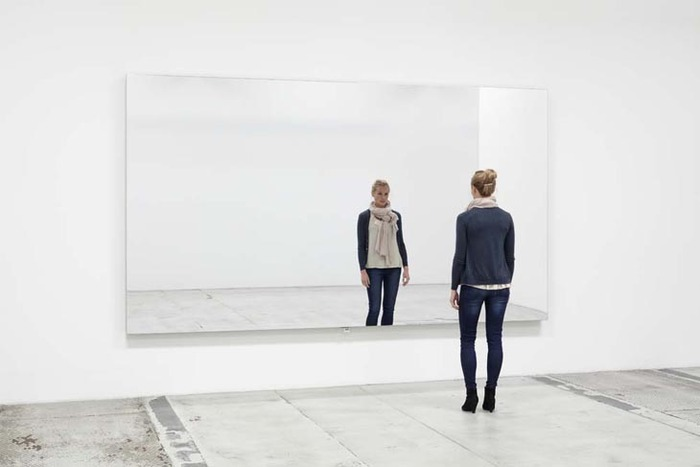Example: In the above image, if you closely look at the lady, you can understand that the left hand of the lady will appear to be her right hand and her right hand will appear as the left hand of the lady. You can try these yourselves by standing in front of a mirror.

## What is Water Image?

A reflection of a particular object in the water is what we call as the water image. A perfect example of a water image can be referred back to a story about a dog with a bone.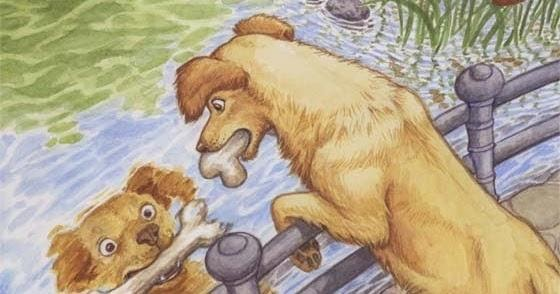In the above image, we can see the exact reflection of the dog with a bone in the river water. This is what we call the water image.

Anita ma'am also cited some problems from previous Olympiads to help them understand from the point of view of reasoning with clear cut solutions.

## Example Problems

Example: In the problem given below, try finding out the water image of the word ‘RADIANT’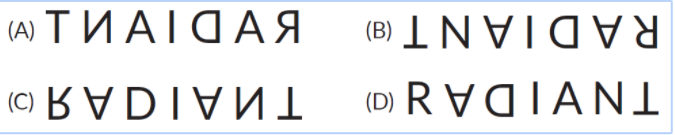Option C

Explanation: The common mistake people do in finding out the water image is that they confuse these questions with those of the mirror images.

We should always remember that the Left-Hand side and the Right-hand side of the image will remain in the same position but the only difference is that the top becomes the bottom and the bottom becomes the top.

Bearing this in mind, in option A, the first image should be the water image of the letter R while the last image should be the water image of the letter ‘T’ which is wrong. In option B, we see the mirror image of the word which is not correct. Let us now consider the other two options C and D.

In option D, the water image of ‘R’ is not correct. Hence the correct option is C.

Example: In the given problem, identify the correct mirror image of the following:

C 6 8 D L A E A P E A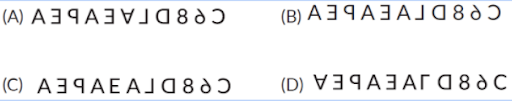Option B

Explanation: For the above problem, let us relate to the rules that

Rule A: LHS and RHS remain in their own positions as their original figure. But our image does not fit into this rule as all the options appear to be correct. Therefore, let us now move on to rule B.

Rule B: In Option A, letter A which comes first in the series seems to be the water image of the original image. Hence option A is not correct. In Option C, the letter ‘E’ appears the same as shown in the original image and so option C is wrong. Option D appears to be the water image of the original image with C reversed and so it is wrong. Hence the correct option is (B)

Example: Identify the mirror image of the given figure if the mirror is to be placed vertically to the right of the given image.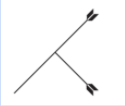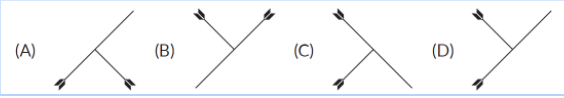Option C

Explanation: In option A, one of the arrows facing downward in the original image is facing upward in the given option and is not correct. In option B, the original image seems to have shifted to the opposite direction and so option B is not correct. In option D, the smaller arrow seems to have shifted above the longer arrow and the longer arrow which was originally facing downward is now facing upwards and this option is also not correct. Hence the correct answer is Option (C)

Example: Imagine that a mirror is placed horizontally at the bottom of the figure as shown below, choose the reflection of the image from the given options.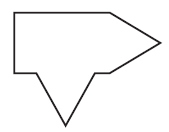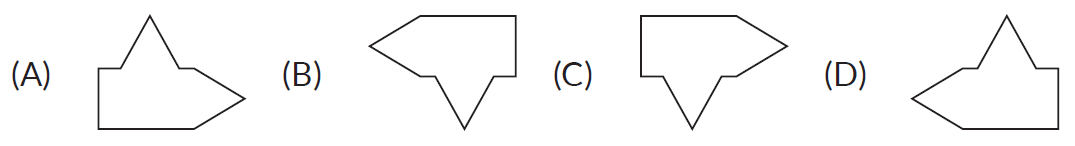Option A

Explanation: The image in option B is the reflection of the object if the mirror is placed near the image and so option B is not the right option. Option C shows the exact image as given in the question and so it is not correct. Option D is the direct opposite figure of its reflection in the mirror and so option D is not correct. Hence as seen closely, option A is the correct option as it is the exact reflection of the image if the mirror is placed below the image.

Example: Identify the water image of the given object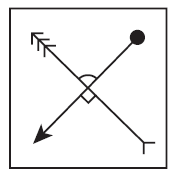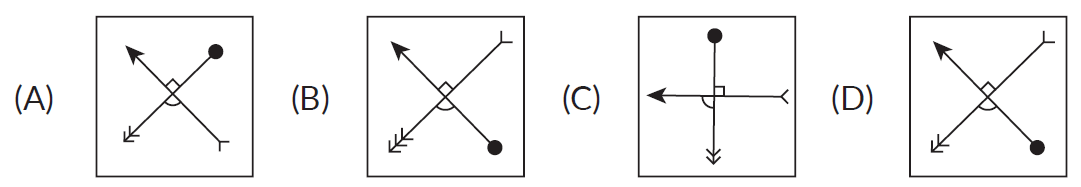Option B

Explanation: If we look at option A, the arrow is correctly facing upward but the ball is on the perpendicular arrow which is not correct. In option C, both the arrows are crossed and the ball is on the wrong arrow and so option C is not correct. In option D, one of the arrows is pointing in the correct side but the arrow which is perpendicular to this arrow is reversed and so option D is not correct. The correct option is B as it is the correct water image of the original figure.

## Practice Problems

1. Identify the reflection of the given image, if the mirror is placed exactly vertical to the right of the given image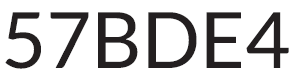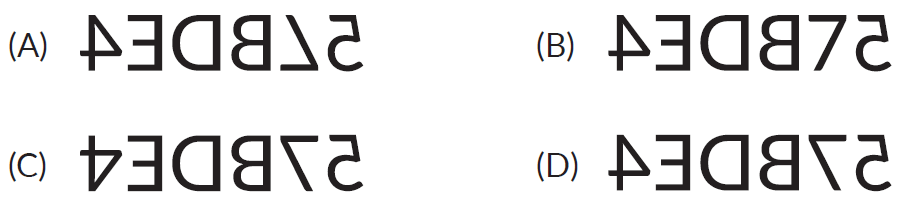2. Identify the mirror image of the given figure if the mirror is placed vertically to the left of the figure.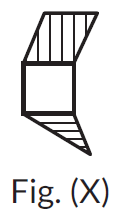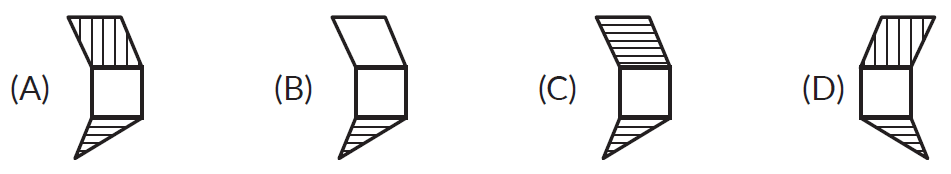4.Identify the correct mirror image of the figure for the following combination of letters and numbers if the mirror is placed along the line MN.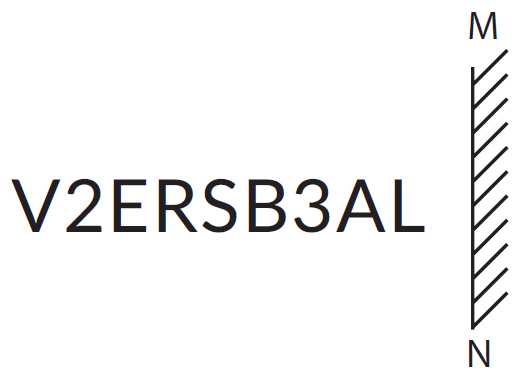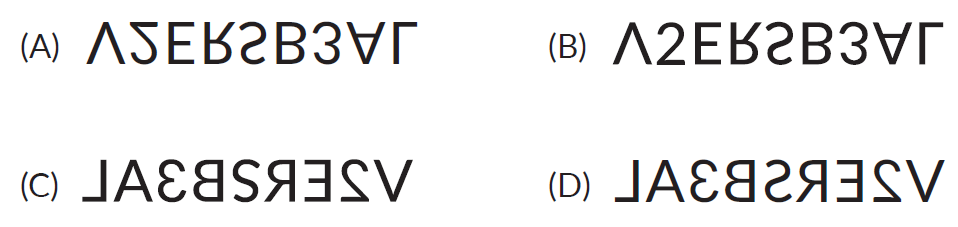1. (D)       2. (A)      3. (D)

## Conclusion

The mirror and water image concept is an important part of the reasoning ability section. in the above article, we learned that the water image is the reflection of an object into the water. It appears upside down by inverting an object vertically. The water image of the figure looks like the mirror image of the figure in case the mirror is horizontally at the bottom of the figure.

Cuemath, a student-friendly mathematics and coding platform, conducts regular Online Classes for academics and skill-development, and their Mental Math App, on both iOS and Android, is a one-stop solution for kids to develop multiple skills. Understand the Cuemath fee structure and sign up for a free trial.

## 1. Is water image and mirror image the same?

The water image is the inverted image obtained by turning the object upside down. The top and bottom part of the image will be changed, while the left and right-hand side of the image remains the same. In the Mirror image left side and right side changed vice-versa where top and bottom remain the same.

## 2. How to calculate mirror and water image of the clock?

To get a mirror image to consider 12 O'clock, which can write 11.60 and subtract the given time from 11.60 to the answer. To get the water image to consider 6.30 (18.30), which can write as 17.90, and subtract the given time to get the answer. A Mirror shows the image with lateral change and Water image of Upside Down.

-Written by Karthika S, Cuemath Teacher

Related Articles